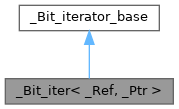ReactOS  0.4.14-dev-50-g13bb5e2
_Bit_iter< _Ref, _Ptr > Struct Template Reference

#include <_bvector.h>

Inheritance diagram for _Bit_iter< _Ref, _Ptr >:[legend]
Collaboration diagram for _Bit_iter< _Ref, _Ptr >:[legend]

Public Types

typedef _Ref reference

typedef _Ptr pointer

typedef _Bit_iter< _Ref, _Ptr > _Self

typedef random_access_iterator_tag iterator_category

typedef bool value_type

typedef ptrdiff_t difference_type

typedef size_t size_typePublic Types inherited from _Bit_iterator_base
typedef ptrdiff_t difference_type

Public Member Functions

_Bit_iter (unsigned int *__x, unsigned int __y)

_Bit_iter ()

_Bit_iter (const _Bit_iter< _Bit_reference, _Bit_reference * > &__x)

reference operator * () const

_Selfoperator++ ()

_Self operator++ (int)

_Selfoperator-- ()

_Self operator-- (int)

_Selfoperator+= (difference_type __i)

_Selfoperator-= (difference_type __i)

_Self operator+ (difference_type __i) const

_Self operator- (difference_type __i) const

difference_type operator- (const _Self &__x) const

reference operator[] (difference_type __i)Public Member Functions inherited from _Bit_iterator_base
void _M_bump_up ()

void _M_bump_down ()

_Bit_iterator_base ()

_Bit_iterator_base (unsigned int *__x, unsigned int __y)

void _M_advance (difference_type __i)

difference_type _M_subtract (const _Bit_iterator_base &__x) constPublic Attributes inherited from _Bit_iterator_base
unsigned int_M_p

unsigned int _M_offset

Detailed Description

template<class _Ref, class _Ptr> struct _Bit_iter< _Ref, _Ptr >

Definition at line 169 of file _bvector.h.

◆ _Self

template<class _Ref, class _Ptr>
 typedef _Bit_iter<_Ref, _Ptr> _Bit_iter< _Ref, _Ptr >::_Self

Definition at line 172 of file _bvector.h.

◆ difference_type

template<class _Ref, class _Ptr>
 typedef ptrdiff_t _Bit_iter< _Ref, _Ptr >::difference_type

Definition at line 175 of file _bvector.h.

◆ iterator_category

template<class _Ref, class _Ptr>
 typedef random_access_iterator_tag _Bit_iter< _Ref, _Ptr >::iterator_category

Definition at line 173 of file _bvector.h.

◆ pointer

template<class _Ref, class _Ptr>
 typedef _Ptr _Bit_iter< _Ref, _Ptr >::pointer

Definition at line 171 of file _bvector.h.

◆ reference

template<class _Ref, class _Ptr>
 typedef _Ref _Bit_iter< _Ref, _Ptr >::reference

Definition at line 170 of file _bvector.h.

◆ size_type

template<class _Ref, class _Ptr>
 typedef size_t _Bit_iter< _Ref, _Ptr >::size_type

Definition at line 176 of file _bvector.h.

◆ value_type

template<class _Ref, class _Ptr>
 typedef bool _Bit_iter< _Ref, _Ptr >::value_type

Definition at line 174 of file _bvector.h.

◆ _Bit_iter() [1/3]

template<class _Ref, class _Ptr>
 _Bit_iter< _Ref, _Ptr >::_Bit_iter ( unsigned int * __x, unsigned int __y )
inline

Definition at line 178 of file _bvector.h.

178 : _Bit_iterator_base(__x, __y) {}

◆ _Bit_iter() [2/3]

template<class _Ref, class _Ptr>
 _Bit_iter< _Ref, _Ptr >::_Bit_iter ( )
inline

Definition at line 179 of file _bvector.h.

179 {}

◆ _Bit_iter() [3/3]

template<class _Ref, class _Ptr>
 _Bit_iter< _Ref, _Ptr >::_Bit_iter ( const _Bit_iter< _Bit_reference, _Bit_reference * > & __x )
inline

Definition at line 181 of file _bvector.h.

◆ operator *()

template<class _Ref, class _Ptr>
 reference _Bit_iter< _Ref, _Ptr >::operator * ( ) const
inline

Definition at line 187 of file _bvector.h.

187  {
188  return _Bit_reference(_M_p, 1UL << _M_offset);
189  }
unsigned int _M_offset
Definition: _bvector.h:107
unsigned int * _M_p
Definition: _bvector.h:106
#define UL
Definition: tui.h:83

◆ operator+()

template<class _Ref, class _Ptr>
 _Self _Bit_iter< _Ref, _Ptr >::operator+ ( difference_type __i ) const
inline

Definition at line 216 of file _bvector.h.

216  {
217  _Self __tmp = *this;
218  return __tmp += __i;
219  }
_Bit_iter< _Ref, _Ptr > _Self
Definition: _bvector.h:172

◆ operator++() [1/2]

template<class _Ref, class _Ptr>
 _Self& _Bit_iter< _Ref, _Ptr >::operator++ ( )
inline

Definition at line 190 of file _bvector.h.

190  {
191  _M_bump_up();
192  return *this;
193  }

◆ operator++() [2/2]

template<class _Ref, class _Ptr>
 _Self _Bit_iter< _Ref, _Ptr >::operator++ ( int )
inline

Definition at line 194 of file _bvector.h.

194  {
195  _Self __tmp = *this;
196  _M_bump_up();
197  return __tmp;
198  }
_Bit_iter< _Ref, _Ptr > _Self
Definition: _bvector.h:172

◆ operator+=()

template<class _Ref, class _Ptr>
 _Self& _Bit_iter< _Ref, _Ptr >::operator+= ( difference_type __i )
inline

Definition at line 208 of file _bvector.h.

208  {
210  return *this;
211  }
Definition: _bvector.h:131

◆ operator-() [1/2]

template<class _Ref, class _Ptr>
 _Self _Bit_iter< _Ref, _Ptr >::operator- ( difference_type __i ) const
inline

Definition at line 220 of file _bvector.h.

220  {
221  _Self __tmp = *this;
222  return __tmp -= __i;
223  }
_Bit_iter< _Ref, _Ptr > _Self
Definition: _bvector.h:172

◆ operator-() [2/2]

template<class _Ref, class _Ptr>
 difference_type _Bit_iter< _Ref, _Ptr >::operator- ( const _Self & __x ) const
inline

Definition at line 224 of file _bvector.h.

224  {
225  return _M_subtract(__x);
226  }
difference_type _M_subtract(const _Bit_iterator_base &__x) const
Definition: _bvector.h:142

◆ operator--() [1/2]

template<class _Ref, class _Ptr>
 _Self& _Bit_iter< _Ref, _Ptr >::operator-- ( )
inline

Definition at line 199 of file _bvector.h.

199  {
200  _M_bump_down();
201  return *this;
202  }
void _M_bump_down()
Definition: _bvector.h:116

◆ operator--() [2/2]

template<class _Ref, class _Ptr>
 _Self _Bit_iter< _Ref, _Ptr >::operator-- ( int )
inline

Definition at line 203 of file _bvector.h.

203  {
204  _Self __tmp = *this;
205  _M_bump_down();
206  return __tmp;
207  }
void _M_bump_down()
Definition: _bvector.h:116
_Bit_iter< _Ref, _Ptr > _Self
Definition: _bvector.h:172

◆ operator-=()

template<class _Ref, class _Ptr>
 _Self& _Bit_iter< _Ref, _Ptr >::operator-= ( difference_type __i )
inline

Definition at line 212 of file _bvector.h.

212  {
213  *this += -__i;
214  return *this;
215  }

◆ operator[]()

template<class _Ref, class _Ptr>
 reference _Bit_iter< _Ref, _Ptr >::operator[] ( difference_type __i )
inline

Definition at line 227 of file _bvector.h.

227 { return *(*this + __i); }

The documentation for this struct was generated from the following file: